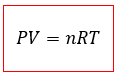# Problem: Calcium carbide reacts with water to produce acetylene gas, C 2H2.CaC2 (s) + 2 H2O (l) → Ca(OH)2 (aq) + C2H2 (g)Calculate the volume (in liters) of acetylene produced at 29°C and 684 mmHg from 0.026 mol CaC 2 and excess H2O.

###### FREE Expert Solution

For this problem, we’re asked to calculate the volume (in liters) of acetylene (C2H2) produced at 29 °C with a pressure of 684 mmHg

The reaction occurs between excess water and 0.026 mol CaC2.

First, remember the ideal gas equationWhere,

P = Pressure (in Atm)

V = Volume (in Liters)

n = number of moles

R = Ideal gas constant (of value 0.08206 atm*L/mol*K)

T = Temperature (in K)

80% (51 ratings)###### Problem Details

Calcium carbide reacts with water to produce acetylene gas, C 2H2.

CaC2 (s) + 2 H2O (l) → Ca(OH)2 (aq) + C2H2 (g)

Calculate the volume (in liters) of acetylene produced at 29°C and 684 mmHg from 0.026 mol CaC 2 and excess H2O.

Frequently Asked Questions

What scientific concept do you need to know in order to solve this problem?

Our tutors have indicated that to solve this problem you will need to apply the Gas Stoichiometry concept. You can view video lessons to learn Gas Stoichiometry. Or if you need more Gas Stoichiometry practice, you can also practice Gas Stoichiometry practice problems.

What professor is this problem relevant for?

Based on our data, we think this problem is relevant for Professor Kobko-Litskevitch's class at HUNTER.